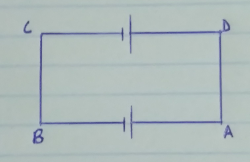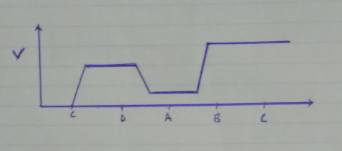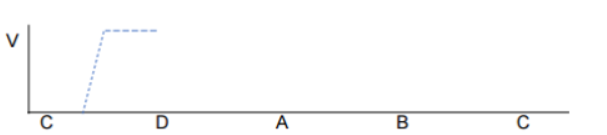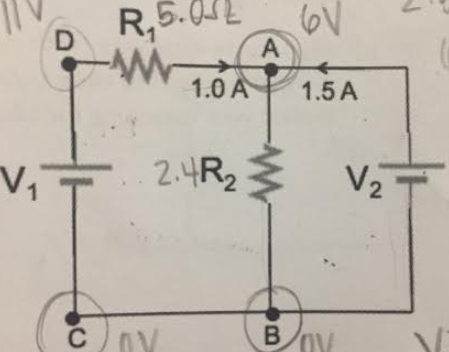# Problem: 2. Sketch, in a plot like the one below, the voltage as one moves around the outer loop in the circuit from part A, from point C through V1 to point D, through R1 to A, through V2 to B, then back to C. Assume point C is at zero volts. Label specific voltages on the y-axis; in other words, the graph should show quantitatively the change in voltages through this loop. The graph has been started for you (dashed line). 3. Explain specifically how one could use the graph above to determine the current flow (direction and magnitude) through resistor R1, which has a resistance of 5 Ω.

###### FREE Expert Solution

1.This cannot be possible since, at B and C, the value of voltage should be the same. This means that the battery was done in the opposite direction.

91% (172 ratings)###### Problem Details

2. Sketch, in a plot like the one below, the voltage as one moves around the outer loop in the circuit from part A, from point C through V1 to point D, through R1 to A, through V2 to B, then back to C. Assume point C is at zero volts. Label specific voltages on the y-axis; in other words, the graph should show quantitatively the change in voltages through this loop. The graph has been started for you (dashed line).3. Explain specifically how one could use the graph above to determine the current flow (direction and magnitude) through resistor R1, which has a resistance of 5 Ω.